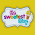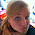### Visual Models for the Division of Fractions

This summer I attended a workshop called Visual Models for the Division of Fractions.  Moving up to 5th grade next year, there's a lot I need to re-teach myself, like dividing fractions!  Eeekkk!  I wanted to share a couple of examples of how to use a visual model to divide fractions.  For each question, we had to draw a linear model or an area model.  I chose a linear model (number line).  Our district Math coach is all about number lines so I figured it would be best for me to try to "see" it that way.

Question #1:
Grandmother has three acres of land.  When she divided it equally among all of her grandchildren, each got 1/2 acre.  How many grandchildren does Grandmother have?

First, I drew a number line and recorded the wholes 0, 1, 2, and 3.  I went up to 3 because there is 3 acres of land. Clearly, I free-handed this (which our presenter was adamant that we don't do...oops!).  After my whole number were recorded, I divided them in half because the grandchildren received 1/2 acres.  Then, I was able to see that there were 6 halves in 3.  So, three wholes divided into halves equal six.
One thing our presenter said was to make students write the answer in word form as well as number form.  Writing in word form helps you see that the child does understand the problem.

Question #2: Four students sitting at a table were given 1/3 of a pan of brownies to share.  How much will each student get if they share the pan equally?

First, I drew a number line to the whole number 1 and split it into thirds since I knew I was dealing with thirds.  Knowing from my problem that 1/3 was being divided into 4 parts, I used a red marker to show this.  Then, I had to find out how much each small part equaled, which is 1/12.  I colored in green to show what part of the number line represents my problem.  Notice though that I still divided the other part of the number line into twelfths.  So, if 4 students share 1/3 a pan of brownies, each student receives 1/12 piece.
Question #3:
We have 1/2 of a gallon of milk to pour equally among 3 pitchers. After pouring, how much will be in each pitcher?

First, I drew my number line to the whole number one and split into half since I have a half gallon of milk.  Knowing the half gallon is split into 3 pitchers, I showed that on my number line as well. Again, note that I split it all the way to the whole.  I didn't just stop at the half mark.  When I did that, I determined that each spot equals 1/6.  So, if we pour 1/2 gallon in 3 pitchers, each pitcher will hold 1/6 gallon.

When drawing visuals models, it really works best if you let students use colored pencils/crayons/pens.  It really helped me to see it by using different colors.

Before going into this workshop, I couldn't even remember how to divide fractions.  Then, I was shown the traditional version where you take one the second fraction and do the inverse and then multiply. So, here I changed the whole number 3 to 1/3 and then multiplied.  Did you notice that this is the same problem as Question #3 above?

But, if I was trying to explain WHY 1/2 divided by 3 equals 1/6 and I did it the traditional way, I wouldn't be able to explain it. Therefore, I wouldn't truly understand it.  Drawing visual models helps students (and teachers!) understand division so much more than using the traditional algorithm.

Now, give me some feedback!  Do you teach division of fractions? If so, what are some ways you teach it?  Would you like to see more sample problems other than the ones shown above?

1.Thank goodness for the sake of my kiddos I don't have to teach math this year. This look like a great idea though!! I had a colleague last year who would post things like this to her blog so parents could use the resource at home to help with HW. This would be great for that!

Lauren
The Sweetest Thing

2.Great tutorial! I'm not going to lie...5th grade math intimidates me! Ha!
Erin
Short and Sassy Teacher

3.Great visuals I bet those will definitely help your students understand the concepts behind dividing fractions better.

Kaylee's Education Studio

4.That sounds like a great workshop! I pinned your post in the event I ever move up to fifth. What a great visual!

Meg
Third Grade in the First State

5.Please do more! I would love to make a PowerPoint presentation on this! More examples the better!

6.